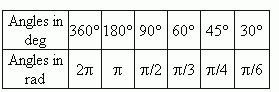# Radian and degree measures of angles

Degree and radian measures of angles.
Relation of a circle radius and a circumference
length. Table of degree and radian measures
for some most used angles.

A degree measure. Here a unit of measurement is a degree (its designation is ° or deg ) a turn of a ray by the  1 / 360   part of the one complete revolution. So, the complete revolution of a ray is equal to 360 deg. One degree is divided into 60 minutes (a designation is ‘ or min ); one minute – correspondingly into  60 seconds (a designation is “ or sec ) .

A radian measure. As we know from plane geometry ( see the point "A length of arc" of the paragraph "Geometric locus. Circle and circumference" ), a length of an arc l , a radius r and a corresponding central angle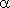are tied by the relation:= l / r .

This formula is a base for definition of a radian measure of angles. So,  if l = r , then= 1, and we say, that an angleis equal to1 radian, that is designed as= 1 rad .  Thus, we have the following definition of a radian measure unit:

A radian is a central angle, for which lengths of  its arc and radius are equal ( A m B = AO, Fig.1 ). So, a radian measure of any angle is a ratio of a length of an arc drawn by an arbitrary radius and concluded between sides of this angle to the arc radius.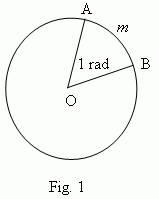Following this formula, a length of a circumference C and its radius r can be expressed as:

2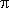= C / r .

So , a round angle, equal to 360° in a degree measure, is simultaneously 2in a radian measure. Hence, we receive a value of one radian: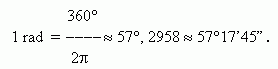Inversely,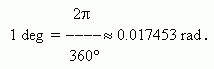It is useful to remember the following comparative table of degree and radian measure for some angles, we often deal with: